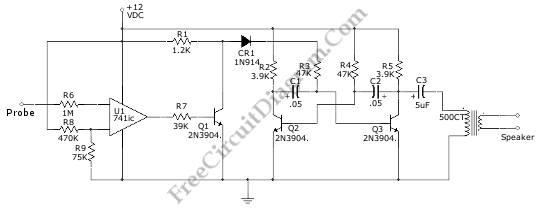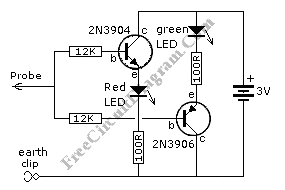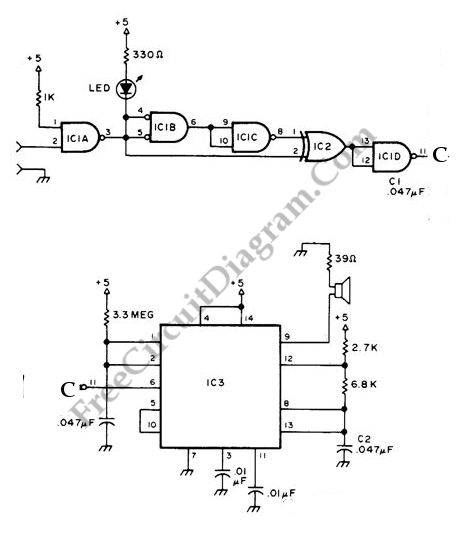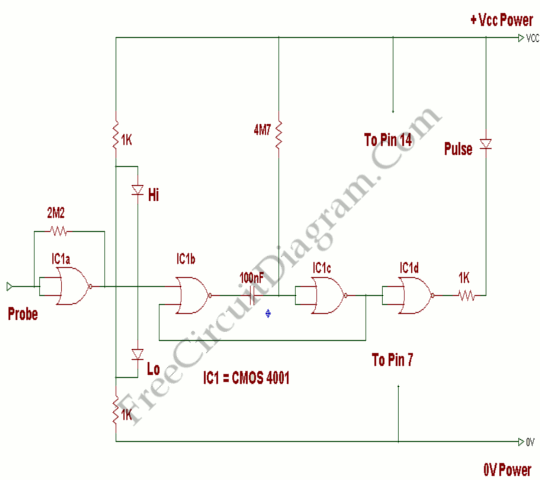## Audible Logic Probe/IndicatorLogic indicator circuit / logic probe is used to identify logic level at any point of logic circuitry. The indicator can be visual (using LED, LCD, of 7 Segments) or auditory (using beeper or speaker). This circuit has a audible indicator, with the help of  a loudspeaker to produce indication tone.  Using the opamp,U1, as schmitt trigger, the Audio oscillator […]

## Simple Discrete Logic ProbeThis is simple logic probe circuit. Logic probe is used to determine if a point in a circuit has high or low state when the circuit is in operation.  Here is the schematic diagram of the circuit: When the probe is not touching any circuitry, this circuit consumes no current. It can happen because the voltage across the red LED […]

## Audible Logic ProbeWe can use NE556 timer IC to make indication of static of terminal in digital logic audible.  Audible logic probe can be very useful when we have to visually inspect a part while need to check the logic state on other point apart from the part.  Here’s the circuit diagram: NE556 will act as oscillator controlled by IC1 (SN74132N) and […]

## Logic Probe with Pulse IndicatorA single CMOS IC is used on this logic probe. This logic probe shows three logic condition, High, Low, and Pulsing. Ind addition, there is no LED’s will glow if the probe input is neither hi or lo ( the high impedance state of tri-output logic IC’s). Here’s the circuit diagram: This logic probe using power from the logic circuit […]